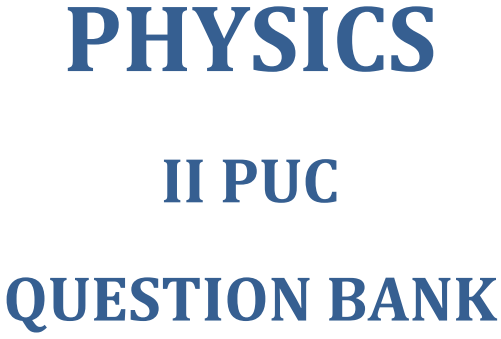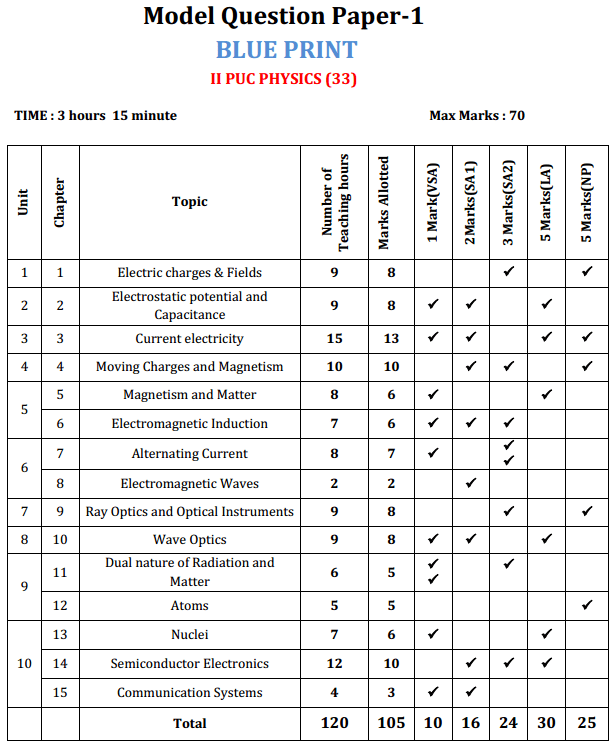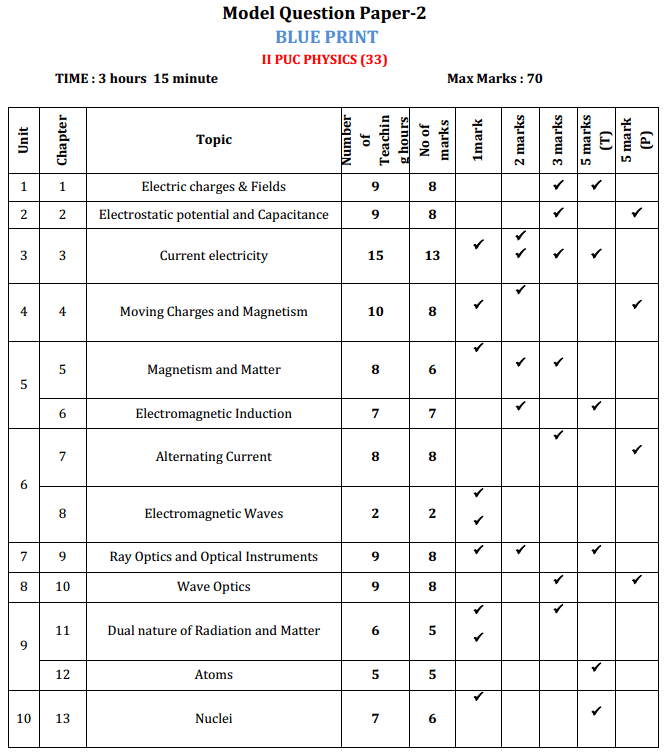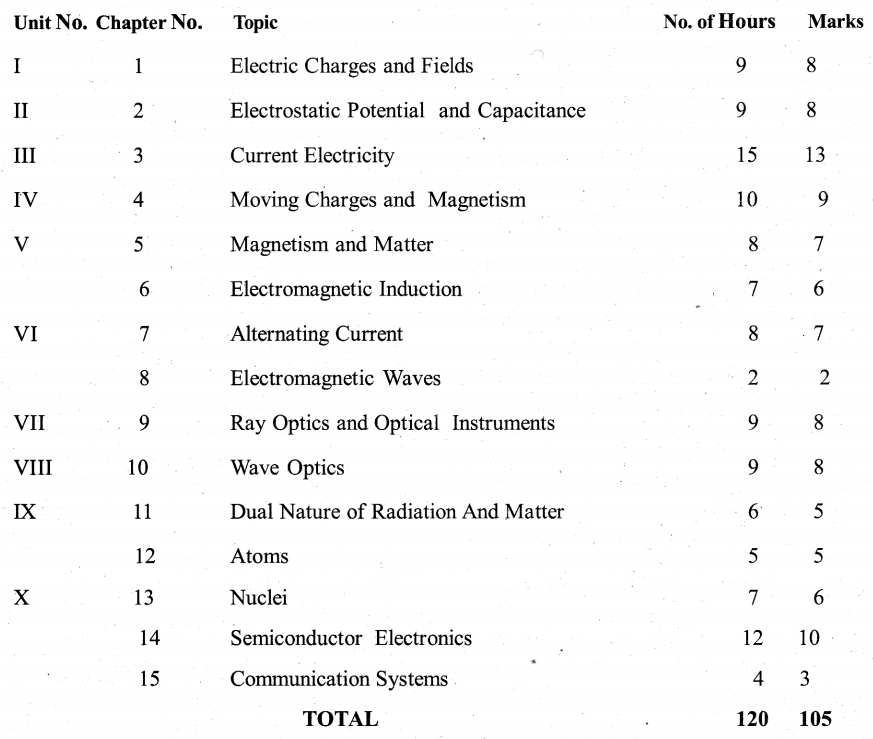# 2nd PUC Physics Question Bank with Answers Karnataka

Expert Teachers at KSEEBSolutions.com has created Karnataka 2nd PUC Physics Question Bank with Answers Solutions, Notes, Guide Pdf Free Download of 2nd PUC Physics Textbook Questions and Answers, Model Question Papers with Answers, Study Material 2020-21 in English Medium and Kannada Medium are part of 2nd PUC Question Bank with Answers. Here KSEEBSolutions.com has given the Department of Pre University Education (PUE) Karnataka State Board NCERT Syllabus 2nd Year PUC Physics Question Bank with Answers Pdf.

Students can also read 2nd PUC Physics Model Question Papers with Answers hope will definitely help for your board exams.## Karnataka 2nd PUC Physics Question Bank with Answers

### Karnataka 2nd PUC Physics Syllabus and Marking Scheme

Karnataka 2nd PUC Physics Blue Print of Model Question PaperKarnataka 2nd PUC Physics Question Paper Design

Time: 3 Hours 15 Minutes (of which 15 minutes for reading the question paper).
Maximum Marks: 70

The weightage of the distribution of marks over different dimensions of the question paper is as follows:

A. Weightage to Objectives:

 Objective Weightage Marks Knowledge 40% 43/105 Understanding 30% 31/105 Application 20% 21/105 Skill 10% 10/105

B. Weightage to Content/Subject Units:C. Weightage to forms of Questions:Note:

1. Questions in IV Main must be set from Unit I to V.
2. Questions in V Main must be set from Unit VI to X.
3. Questions in VI Main must be set such that one Numerical Problem is from every 2 successive units.

D. Weightage to the Level of Difficulty:

 Level Weightage Marks Easy 40% 43/105 Average 40% 42/105 Difficult 20% 20/105

General Instructions

• Questions should be clear, unambiguous, understandable and free from grammatical errors.
• Questions which are based on the same concept, law, fact etc. and which generate the same answer should not be repeated under different forms (VSA, SA, LA and NP).
• Questions must be set based on the blowup syllabus only.

2nd PUC Physics Practical Exam Syllabus

List of Experiments

1. To find resistance of a given wire using metre bridge and hence determine the specific resistance of its material.
2. To determine resistance per cm of a given wire .by plotting a graph of potential difference versus current.
3. To verify the laws of combination (series/ parallel) of resistances using a metre bridge.
4. To compare the EMFs of two given primary cells using potentiometer.
5. To determine the internal resistance of given primary cell using potentiometer.
6. To determine resistance of a galvanometer by half-deflection method and to find its.figure of merit.
7. To convert the given galvanometer (of known resistance of figure of merit) into an ammeter and voltmeter of desired range and to verify the same.
8. To find the frequency of the ac mains with a sonometer.
9. To find the value of v for different values of u in case of a concave mirror and to find the focal ‘ length.
10. To find the focal length of a convex mirror, using a convex lens.
11. To find the focal length of a convex lens by plotting graphs between u and v or between 1/uand 1/v.
12. To find the focal length of a concave lens, using a convex lens.
13. To determine angle of minimum deviation for a given prism by plotting a graph between the angle of incidence and the angle of deviation.
14. To determine the refractive index of a glass slab using a travelling microscope.
15. To find the refractive index of a liquid by using
• Concave mirror
• Convex lens and plane mirror.
16. To draw the I-V characteristics curves of a p-n junction in forward bias and reverse bias.
17. To draw the characteristics curve of a Zener diode and to determine its reverse break down voltage.
18. To study the characteristics of a common- emitter NPN or PNP transistor and to find out the values of current and voltage gains.

2nd PUC Physics Practical Exam Marking Scheme

General Instructions

• Duration of practical examination: 2 hours.
• Maximum marks allotted: 30 marks.
• At least TEN (10) different experiments have to be set in the practical Examination.

Scheme of Evaluation

A. Weightage of Marks

 S.No. Particulars Marks I Performing the Experiment 20 II Viva – Voce 4 III Practical Record 6 Total 30

B. Distribution of Marks

I. Performing the Experiment

1. Writing the principle of the experiment (2 Marks)
2. Writing the formula and explaining the terms (2 Marks)
3. Writing the diagram/figure/circuit with labelling (At least two parts) (2 Marks)
4. Writing the tabular column/observation pattern (2 Marks)
5. Constructing the experimental set up/ circuit (3 Marks)
6. Performing the experiment and entering the readings into the tabular column/Observation pattern (4 Marks)
7. Substitution and calculation/plotting the graph and calculation (3 Marks)
8. Result with a unit (2 Marks)
9. Total 20 marks

Note for S.No.6

• At least three (3) trials have to be taken in case of finding mean value.
• At least six (6) readings have to be taken in case of plotting the graph.

II. Viva – Voce

1. Four questions must be asked and each question carries 1 mark.
2. The questions in the viva- voce should be simple, direct and related to the experiment being performed by the student.

III. Practical Record

1. If the student has performed and recorded 13 experiments or more (6 Marks)
(91% to 100% of the experiments prescribed for the practical examination or more)
2. If the student has performed and recorded 11 or 12 experiments. (5 Marks)
(81% to 90% of the experiments prescribed for the practical examination)
3. If the student has performed and recorded 10 experiments. (4 Marks)
(71% to 80% of the experiments prescribed for the practical examination)
4. If the student has performed and recorded below 10 and above 5 experiments. (3 Marks)
(41% to 70% of the experiments prescribed for the practical examination)
5. If die student has performed and recorded 5 or less than 5 experiments. (0 Marks)
(40% & below 40% Of the experiments prescribed for the practical examination)

We hope the given Karnataka 2nd PUC Class 12 Physics Question Bank with Answers Solutions, Notes, Guide Pdf Free Download of 2nd PUC Physics Textbook Questions and Answers, Model Question Papers with Answers, Study Material 2020-2021 in English Medium and Kannada Medium will help you.

If you have any queries regarding Karnataka State Board NCERT Syllabus 2nd Year PUC Class 12 Physics Question Bank with Answers Pdf, drop a comment below and we will get back to you at the earliest.

error: Content is protected !!